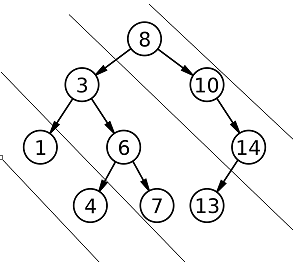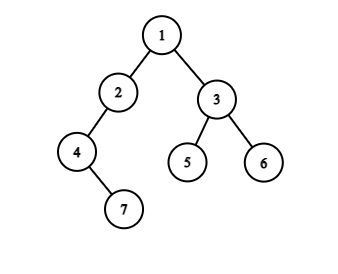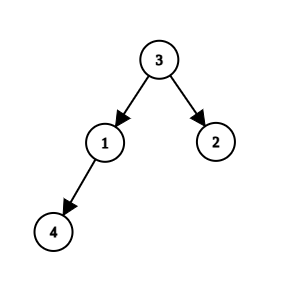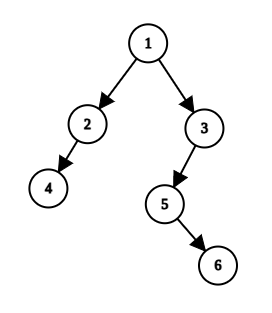5

# Diagonal Traversal

Difficulty: MEDIUM
Avg. time to solve
15 min
Success Rate
85%

Problem Statement
Suggest Edit

#### A binary tree is a hierarchical data structure in which each node has at most two children.

##### For Example:``````For the above binary tree, the output will be:
8 10 14
3 6 7 13
1 4
``````
##### Input Format:
``````The first line contains an Integer 'T' which denotes the number of test cases or queries to be run. Then the test cases follow.

The first line of each test case contains the elements of the tree in the level order form separated by a single space.
If any node does not have a left or right child, take -1 in its place. Refer to the example below.
``````
##### Example:
``````Elements are in the level order form. The input consists of values of nodes separated by a single space in a single line. In case a node is null, we take -1 in its place.

For example, the input for the tree depicted in the below image would be :
````````````1
2 3
4 -1 5 6
-1 7 -1 -1 -1 -1
-1 -1

Explanation :
Level 1 :
The root node of the tree is 1

Level 2 :
Left child of 1 = 2
Right child of 1 = 3

Level 3 :
Left child of 2 = 4
Right child of 2 = null (-1)
Left child of 3 = 5
Right child of 3 = 6

Level 4 :
Left child of 4 = null (-1)
Right child of 4 = 7
Left child of 5 = null (-1)
Right child of 5 = null (-1)
Left child of 6 = null (-1)
Right child of 6 = null (-1)

Level 5 :
Left child of 7 = null (-1)
Right child of 7 = null (-1)

The first not-null node (of the previous level) is treated as the parent of the first two nodes of the current level. The second not-null node (of the previous level) is treated as the parent node for the next two nodes of the current level and so on.

The input ends when all nodes at the last level are null (-1).

Note: The above format was just to provide clarity on how the input is formed for a given tree.

The sequence will be put together in a single line separated by a single space. Hence, for the above-depicted tree, the input will be given as:

1 2 3 4 -1 5 6 -1 7 -1 -1 -1 -1 -1 -1
``````
##### Output Format :
``````For each test case, print each diagonal traversal in a new line.
The output of each test case should be printed in a separate line.
``````
##### Note
``````You are not required to print anything, it has already been taken care of. Just implement the function.
``````
##### Constraints:
``````1 <= T <= 100
1 <= N <= 3 * 10^3
1 <= nodeVal <= 10^9

Time Limit: 1 sec.
``````
##### Sample Input 1:
``````2
3 1 2 4 -1 -1 -1 -1 -1
1 2 3 4 -1 5 -1 -1 -1 -1 6 -1 -1
``````
##### Sample Output 1:
``````3 2
1
4
1 3
2 5 6
4
``````
##### Explanation For Sample Output 1:
``````For the first test case, the tree will be:
````````````So, the output will be:
3 2
1
4

For the second test case, the tree will be:
````````````So, the output will be:
1 3
2 5 6
4
``````
##### Sample Input 2:
``````2
1 2 -1 -1 -1
1 3 2 -1 -1 -1 -1
``````
##### Sample Output 2:
``````1
2
1 2
3
``````Console